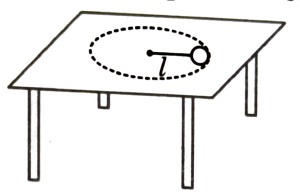Dynamics of uniform and non uniform circular motion
Question

# A body is moving on a rough horizontal table in a circular path being tied to a nail by a string. While the body is in motion, match the quantities in column-A with their description in column-B.Column-AColumn-B(a)Acceleration of body(p)Changes direction but constant in magnitude(b)Friction force on body(q)Changes magnitude but constant in direction(c)Angular momentum of the particle(r)Changes direction as well as magnitude(d)Normal force by table(s)Direction as well as the magnitude is constant

Moderate
Solution

## Due to friction${\mathrm{a}}_{\mathrm{t}}=\mathrm{\mu g}$ (Kinetic friction will act opposite to velocity)${\mathrm{a}}_{\mathrm{r}}=\frac{{\mathrm{v}}^{2}}{\mathrm{R}}$(a) Magnitude of ar changes because speed changes so magnitude and direction (always remaining towards centre) both changes.(b) Kinetic friction is constant in magnitude but direction is opposite to velocity.(c) Angular momentum decreases in magnitude but remains in same direction.(d) N remains constant in magnitude and direction.

Get Instant Solutions